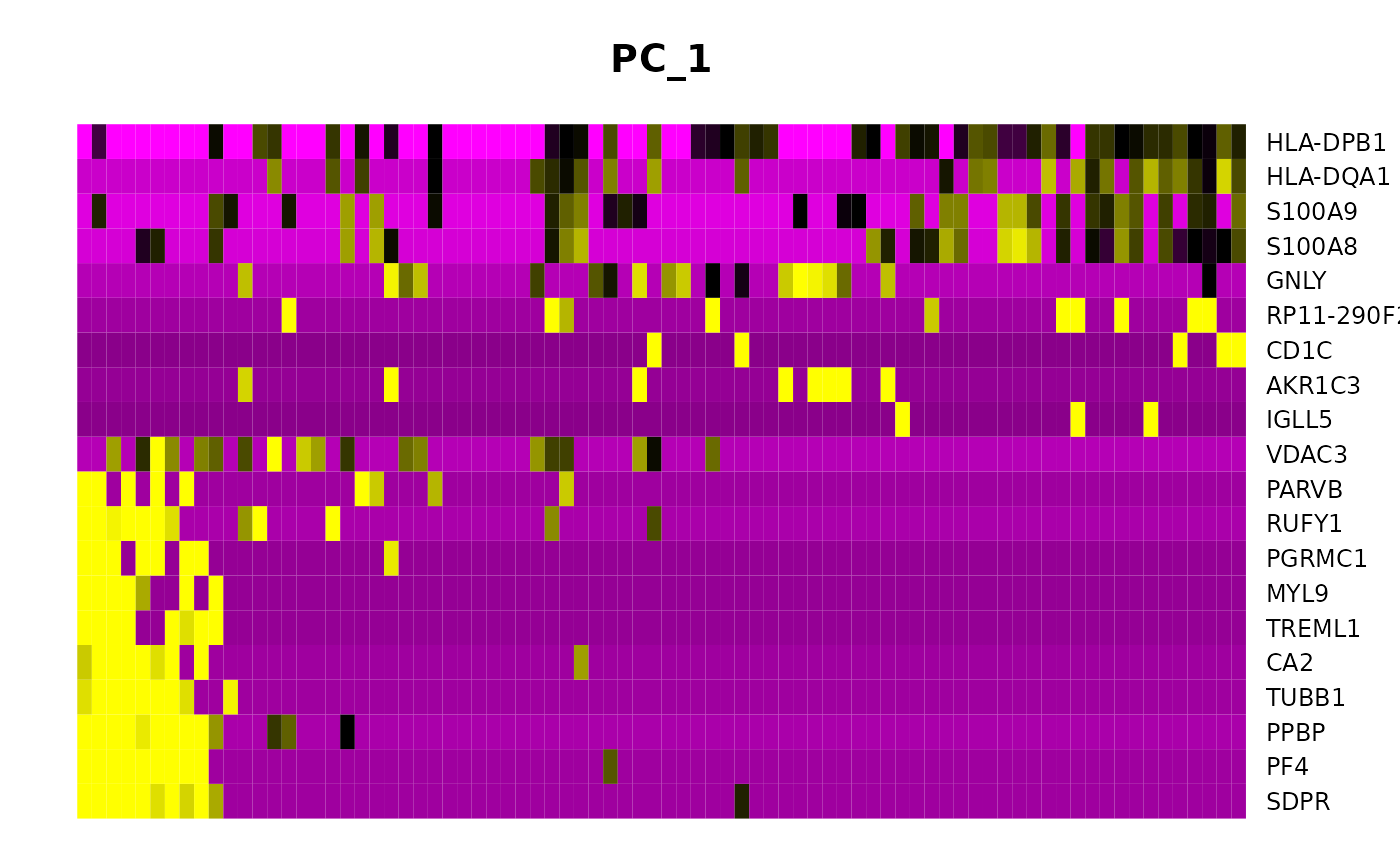Draws a heatmap focusing on a principal component. Both cells and genes are sorted by their principal component scores. Allows for nice visualization of sources of heterogeneity in the dataset.

DimHeatmap(
object,
dims = 1,
nfeatures = 30,
cells = NULL,
reduction = "pca",
disp.min = -2.5,
disp.max = NULL,
balanced = TRUE,
projected = FALSE,
ncol = NULL,
fast = TRUE,
raster = TRUE,
slot = "scale.data",
assays = NULL,
combine = TRUE
)

PCHeatmap(object, ...)

## Arguments

object

Seurat object

dims

Dimensions to plot

nfeatures

Number of genes to plot

cells

A list of cells to plot. If numeric, just plots the top cells.

reduction

Which dimensional reduction to use

disp.min

Minimum display value (all values below are clipped)

disp.max

Maximum display value (all values above are clipped); defaults to 2.5 if slot is 'scale.data', 6 otherwise

balanced

Plot an equal number of genes with both + and - scores.

projected

Use the full projected dimensional reduction

ncol

Number of columns to plot

fast

If true, use image to generate plots; faster than using ggplot2, but not customizable

raster

If true, plot with geom_raster, else use geom_tile. geom_raster may look blurry on some viewing applications such as Preview due to how the raster is interpolated. Set this to FALSE if you are encountering that issue (note that plots may take longer to produce/render).

slot

Data slot to use, choose from 'raw.data', 'data', or 'scale.data'

assays

A vector of assays to pull data from

combine

Combine plots into a single patchworked ggplot object. If FALSE, return a list of ggplot objects

...

Extra parameters passed to DimHeatmap

## Value

No return value by default. If using fast = FALSE, will return a patchworked ggplot object if combine = TRUE, otherwise returns a list of ggplot objects

image geom_raster
data("pbmc_small")